Test: Thyristor Chopper Circuits

# Test: Thyristor Chopper Circuits

Test Description

## 5 Questions MCQ Test Power Electronics | Test: Thyristor Chopper Circuits

Test: Thyristor Chopper Circuits for Electrical Engineering (EE) 2023 is part of Power Electronics preparation. The Test: Thyristor Chopper Circuits questions and answers have been prepared according to the Electrical Engineering (EE) exam syllabus.The Test: Thyristor Chopper Circuits MCQs are made for Electrical Engineering (EE) 2023 Exam. Find important definitions, questions, notes, meanings, examples, exercises, MCQs and online tests for Test: Thyristor Chopper Circuits below.
Solutions of Test: Thyristor Chopper Circuits questions in English are available as part of our Power Electronics for Electrical Engineering (EE) & Test: Thyristor Chopper Circuits solutions in Hindi for Power Electronics course. Download more important topics, notes, lectures and mock test series for Electrical Engineering (EE) Exam by signing up for free. Attempt Test: Thyristor Chopper Circuits | 5 questions in 15 minutes | Mock test for Electrical Engineering (EE) preparation | Free important questions MCQ to study Power Electronics for Electrical Engineering (EE) Exam | Download free PDF with solutions
 1 Crore+ students have signed up on EduRev. Have you?
Test: Thyristor Chopper Circuits - Question 1

### In a 110 V DC chopper drive using the CLC scheme, the maximum possible value of the accelerating current is 300 A. The lower limit of the current pulsation is 140 A. What is the maximum limit of current pulsation?

Detailed Solution for Test: Thyristor Chopper Circuits - Question 1

Current Limit Control of Chopper (CLC):
In this strategy, the chopper is turned on when the output current i0 equals a preset value I2.
The chopper is kept on till i0 increases to another preset value I1.
The chopper is turned off when i0 equals I1 and is kept off till the current i0 decays to I2.
The current i0 is thus limited to a maximum of I1 and a minimum of I2 as shown in Figure.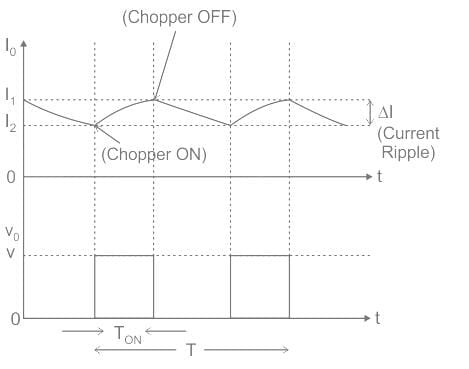The chopping frequency and the pulse width are dependent on the load parameters.
The advantage of current limit control is that the ripple (I1 - I2), in the load current i0 can be adjusted to a small, predetermined value for a wide range of loads.
Application:
Given,
The maximum possible value of the accelerating current (I2) = 300 A
Current pulsation or current ripple (ΔI) = 140 A
The waveform can be drawn as,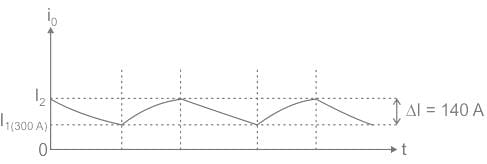From the waveform,
The maximum limit of current pulsation (I2) = I1 + ΔI = 300 + 140 = 440 A

Test: Thyristor Chopper Circuits - Question 2

### In the LC circuit shown in the figure initial current through the inductor is zero, initial voltage across the capacitor is 100 V. Switch S is closed at t = 0 sec. The current through the circuit is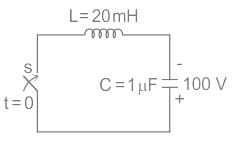Detailed Solution for Test: Thyristor Chopper Circuits - Question 2

Concept:
In a LC circuit, the current flows in the circuit is given by,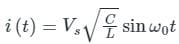Where, VS­ is supply voltage in V
C is capacitance in F
L is inductance in H
ω0 is resonant frequency in rad/sec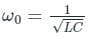Calculation:
From the given circuit, VS = 100 V
C = 1 μF
L = 20 mH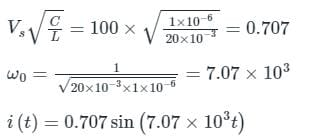*Answer can only contain numeric values
Test: Thyristor Chopper Circuits - Question 3

### In the circuit shown in figure find the circuit turn off time is ______ μs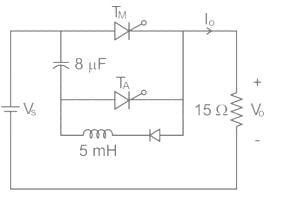Detailed Solution for Test: Thyristor Chopper Circuits - Question 3

In voltage commutation circuit
Circuit turn off time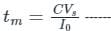for highly inductive load
tm = RC ln 2 ------- for resistive load
∴ tm = 15 × 8 × 10-6 ln 2 = 83.18 μs

Test: Thyristor Chopper Circuits - Question 4

Consider the circuit shown below: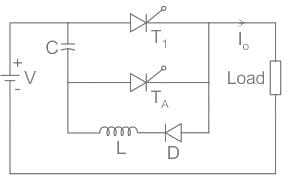The effective on period of the chopper if V =230 V,Io = 60 A,C = 55 μF,Ton = 800 μs is:

Detailed Solution for Test: Thyristor Chopper Circuits - Question 4

Concept:
Mode 1: T1 will remain ON and conduct to load and diode TA will remain OFF
Mode 2: TA will be turned ON; the capacitor current will now flow in reverse direction & T1 stops conducting.
Therefore, the effective time period of the chopper is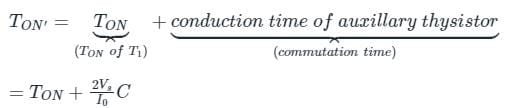Commutation time: It is the time taken to disconnect the load from the supply after the main thyristor is turned OFF.
Calculation:
Effective on period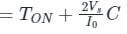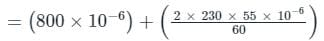= (0.8 + 0.42) × 10-3
TON’ = 1.22 ms

*Answer can only contain numeric values
Test: Thyristor Chopper Circuits - Question 5

What value of capacitor (in μF) is required to forces commutate a thyristor with a turn off time of 20 μs with a 96 V battery and a full load current of 100 A.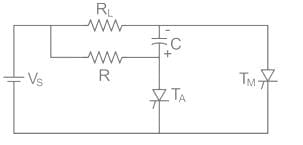Detailed Solution for Test: Thyristor Chopper Circuits - Question 5

The size of capacitor enquired for commutation is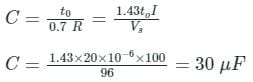## Power Electronics

5 videos|39 docs|63 tests
 Use Code STAYHOME200 and get INR 200 additional OFF Use Coupon Code
Information about Test: Thyristor Chopper Circuits Page
In this test you can find the Exam questions for Test: Thyristor Chopper Circuits solved & explained in the simplest way possible. Besides giving Questions and answers for Test: Thyristor Chopper Circuits, EduRev gives you an ample number of Online tests for practice

## Power Electronics

5 videos|39 docs|63 tests